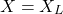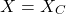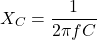# Electrical Reactance: What is it? (Inductive & Capacitive)

Contents

## What is Reactance?

Reactance (also known as electrical reactance) is defined as the opposition to the flow of current from a circuit element due to its inductance and capacitance. Greater reactance leads to smaller currents for the same applied voltage. Reactance is similar to electric resistance, although it differs in several respects.

When an alternating current passes through the electric circuit or element, the phase, and amplitude of current will change. The reactance is used to compute this change in phase and magnitude of the current and voltage waveforms.

When an alternating current passes through the element, the energy is stored in the element that contains reactance. The energy is released in the form of an electric field or magnetic field. In the magnetic field, the reactance resists change in current, and in the electric field, it resists the change in voltage.

The reactance is inductive if it releases energy in the form of a magnetic field. And the reactance is capacitive if it releases energy in the form of an electric field. As frequency increases, capacitive reactance decreases, and inductive reactance increases.

An ideal resistor has zero reactance, whereas ideal inductors and capacitors have zero resistance.

### Reactance Formula

The reactance is denoted as ‘X’. Total reactance is a summation of inductive reactance (XL) and capacitive reactance (XC).When a circuit element contains only inductive reactance, the capacitive reactance is zero and total reactance;When the circuit element contains only capacitive reactance, the inductive reactance is zero and total reactance;The unit of reactance is similar to the unit of resistance and impedance. The reactance is measured in Ohm (Ω).

## What is Inductive Reactance?

The inductive reactance is defined as the reactance produces due to the inductive element (inductor). It is denoted as XL. The inductive elements are used to temporarily store electrical energy in the form of a magnetic field.

When an alternating current passes through the circuit, the magnetic field creates around it. The magnetic field is changing as a result of the current.

Change in the magnetic field induces another electric current in the same circuit. According to the Lenz law, the direction of this current is opposite to the main current.

Hence, the inductive reactance opposes the change of current through the element.

Due to the inductive reactance, the current flow results in the delay and it will create the phase difference between current and voltage waveforms. For the inductive circuit, the current lags the voltage.

For an ideal inductive circuit, the current lags voltage by 90˚. Due to the inductive reactance, the power factor is lagging. The phasor diagram for the ideal inductive circuit is as shown in the below figure.

### Inductive Reactance Formula

The inductive reactance is directly proportional to the frequency. Therefore, if the frequency increases, the inductive reactance increases.

The inductive reactance depends on the supply frequency and the inductance of that element. The formula of inductive reactance is;### Unit of Inductive Reactance

The unit of inductive reactance is a similar unit to the reactance and that is OHM (Ω).

## What is Capacitive Reactance?

The capacitive reactance is defined as the reactance produced due to the capacitive elements (Capacitor). It is denoted as XC. It is an opposition of voltage across the capacitive element.

The capacitive elements are used to temporarily store electrical energy in the form of an electric field.

Due to the capacitive reactance, create a phase difference between the current and voltage. For the capacitive circuit, the current leads the voltage. For the ideal capacitive circuit, the current leads the voltage by 90˚. Due to capacitive reactance, a power factor of the system or circuit is leading. The phasor diagram for the ideal capacitance circuit is as shown in the below figure.

### Capacitive Reactance Formula

Capacitive reactance is inversely proportional to the supply frequency and the capacitance of that element. Therefore, if the supply frequency increases, the capacitance is decreased. The capacitance formula is as shown in the below equation.### Unit of Capacitive Reactance

The unit of capacitive reactance is OHM (Ω).

## Reactance vs Impedance

The reactance (X) is a part of impedance (Z). The below table shows the comparison between both identical terms.

## Reactance vs Resistance

The below table shows a comparison between Reactance and Resistance.

## Reactance of Transmission Line

In an electrical power system, the transmission line is the best example to learn the reactance. Because it has both the reactance; inductive reactance as well as capacitive reactance.

The transmission line is also considered as LC circuit which has inductance and capacitance. Due to the reactance of the transmission line, the voltage and current are not in phase. There is a phase difference. This phase different causes power loss in the form of reactive power.

In a power system network, most of the load is inductive in nature. Therefore, to reduce the phase angle between current and voltage waveforms, the capacitor or other compensation techniques are used to keep the phase difference as low as possible.

Due to inductive nature, the power factor of transmission is lagging in most of the conditions. When a transmission line loaded lightly, in this condition, the power factor is near the unity.

Want To Learn Faster? 🎓
Get electrical articles delivered to your inbox every week.
No credit card required—it’s 100% free.